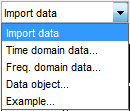## Import Time-Domain Data into the App

Before you can import time-domain data into the System Identification app, you must import the data into the MATLAB® workspace, as described in Time-Domain Data Representation.

Note

Your time-domain data must be sampled at equal time intervals. The input and output signals must have the same number of data samples.

To import data into the app:

1. Type the following command in the MATLAB Command Window to open the app:

`systemIdentification`
2. In the System Identification app window, select Import data > Time domain data. This action opens the Import Data dialog box.3. Specify the following options:

Note

For time series, only import the output signal and enter `[]` for the input.

• Input — Enter the MATLAB variable name (column vector or matrix) or a MATLAB expression that represents the input data. The expression must evaluate to a column vector or matrix.

• Output — Enter the MATLAB variable name (column vector or matrix) or a MATLAB expression that represents the output data. The expression must evaluate to a column vector or matrix.

• Data name — Enter the name of the data set, which appears in the System Identification app window after the import operation is completed.

• Starting time — Enter the starting value of the time axis for time plots.

Tip

The System Identification Toolbox™ product uses the sample time during model estimation and to set the horizontal axis on time plots. If you transform a time-domain signal to a frequency-domain signal, the Fourier transforms are computed as discrete Fourier transforms (DFTs) using this sample time.

4. (Optional) In the Data Information area, click to expand the dialog box and enter the following settings:

Input Properties

• InterSample — This options specifies the behavior of the input signals between samples during data acquisition. It is used when transforming models from discrete-time to continuous-time and when resampling the data.

• `zoh` (zero-order hold) indicates that the input was piecewise-constant during data acquisition.

• `foh` (first-order hold) indicates that the output was piecewise-linear during data acquisition.

• `bl` (bandwidth-limited behavior) specifies that the continuous-time input signal has zero power above the Nyquist frequency (equal to the inverse of the sample time).

Note

See the `d2c` and `c2d` reference pages for more information about transforming between discrete-time and continuous-time models.

• Period — Enter `Inf` to specify a nonperiodic input. If the underlying time-domain data was periodic over an integer number of periods, enter the period of the input signal.

Note

If your data is periodic, always include a whole number of periods for model estimation.

Channel Names

• Input — Enter the name of one or more input channels.

Tip

Naming channels helps you to identify data in plots. For multivariable input-output signals, you can specify the names of individual Input and Output channels, separated by commas.

• Output — Enter the name of one or more output channels.

Physical Units of Variables

• Input — Enter the input units.

Tip

When you have multiple inputs and outputs, enter a comma-separated list of Input and Output units corresponding to each channel.

• Output — Enter the output units.

Notes — Enter comments about the experiment or the data. For example, you might enter the experiment name, date, and a description of experimental conditions. Models you estimate from this data inherit your data notes.

5. Click . This action adds a new data icon to the System Identification app window.

6. Click to close the Import Data dialog box.

## SupportGet trial now